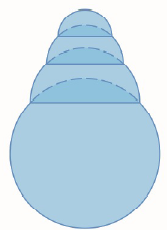Chapter 3.P, Problem 24P

Chapter
Section
Textbook Problem

# A hemispherical bubble is placed on a spherical bubble of radius 1. A smaller hemispherical bubble is then placed on the first one. This process is continued until n chambers, including the sphere, are formed. (The figure shows the case n = 4 .) Use mathematical induction to prove that the maximum height of any bubble tower with n chambersis 1 + n .To determine

To prove:

The maximum height of any bubble tower with n chambers is 1+n

Explanation

1) Concept:

i. Use mathematical induction to prove the statement.

ii. Use the second derivative test to get whether critical number stands for maxima or minima. If c is critical number of a function f and it is continuous at that point, then if f''c>0,  then at x=c; the function has local minima. And f''c<0,  then at x=c, the function has local maxima.

2) Given:

Bubble tower with n chambers and the base is a spherical bubble with radius 1.

3) Calculation:

Prove this by using induction.

By ignoring the bottom of the hemisphere of the initial spherical bubble, we need to prove

“The maximum height of a heap of n hemispherical bubbles is n if the radius of the bottom hemisphere is 1.”

Proceed by induction.

When n=1, then the height of the first hemisphere will be 1.

So the given statement is true for base step.

Suppose this is true for n=k.

So, the maximum height of a heap of k hemispherical bubbles is k if the radius of the bottom hemisphere is 1.

Now suppose there are k+1 hemispherical bubbles forming the stack of maximum height.

Now consider the second bubble having radius r. then the height of the heap formed by the k bubbles is k ·r

The height of the first bubble shown above is 1-r2 using the Pythagorean theorem for that triangle.

Therefore, the height of whole heap is

Hr=kr+1-r2

Here it is needed to choose this value of r in such a way that the height Hr will be maximum.

But since bubbles are placed on each other, we have 0<r<1.

Differentiating Hr with respect to r,H'r=k+r1-r2

H''r=-11-r23/2

To find the critical points, equate H'r to 0

### Still sussing out bartleby?

Check out a sample textbook solution.

See a sample solution

#### The Solution to Your Study Problems

Bartleby provides explanations to thousands of textbook problems written by our experts, many with advanced degrees!

Get Started

#### In problems 1-4, solve the equations 102(2x9)4(6+x)=52

Mathematical Applications for the Management, Life, and Social Sciences

#### Evaluate the integral. 14(4+6uu)du

Single Variable Calculus: Early Transcendentals

#### True or False: y′ + xey = ex+y is separable.

Study Guide for Stewart's Single Variable Calculus: Early Transcendentals, 8th